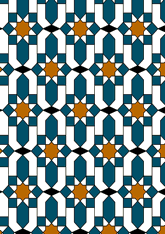data173/MOR0901

## Geometry

• The symmetry group of the tiling is 2*22 (cmm).
• All the internal angles of the constituent polygons are a multiple of 22.5°.
• Contains one square.
• Contains one regular two-pointed star polygon with vertex angle of 45°.
• Contains one regular 8-pointed star polygon with vertex angle of 90°.
• There are two non-regular reflective tiles (including one kite) and one reflective pair.
• The tiling does not satisfy the two-colour condition.
• The tiling is not edge-to-edge.
• As drawn, contains about 573 polygons.

## References

Publications referenced: